1:32 AM A Vectorial Approach: Hamilton's Equation and the Runge Lenz Vector A Vectorial Approach: Hamilton's Equation and the Runge Lenz Vector (Mainly following Milne, Vectorial Mechanics, p 235 on.) Laplace and Hamilton developed a rather different approach to this inverse-square orbit problem, best expressed vectorially, and made a surprising discovery: even though conservation of angular momentum and of energy were enough to determine the motion completely, for the special case of an inverse-square central force, something else was conserved. So the system has another symmetry! Hamilton's approach (actually vectorized by Gibbs) was to apply the operator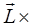to the equation of motion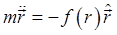: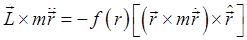Now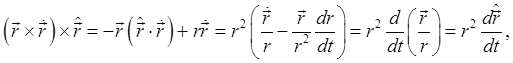so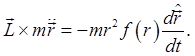This is known as Hamilton's equation. In fact, it's pretty easy to understand on looking it over: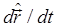has magnitude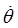and direction perpendicular to,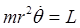, etc. It isn't very useful, though -- except in one case, the inverse-square: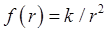(so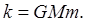) Then it becomes tractable: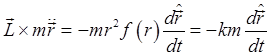and -- surprise -- this integrates immediately to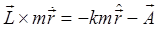whereis a vector constant of integration, that is to say we find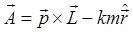is constant throughout the motion! This is unexpected: we found the usual conserved quantities, energy and angular momentum, and indeed they were sufficient for us to find the orbit. But for the special case of the inverse-square law, something else is conserved. It's called the Runge Lenz vector (sometimes Laplace Runge Lenz, and in fact Runge and Lenz don't really deserve the fame -- they just rehashed Gibbs' work in a textbook). From our earlier discussion, this conserved vector must correspond to a symmetry. Finding the orbit gives some insight into what's special about the inverse-square law. Deriving the Orbital Equation from the Runge-Lenz Vector The Runge Lenz vector gives a very quick derivation of the elliptic orbit, without Bernoulli's unobvious tricks in the standard derivation presented above. First, taking the dot product ofwith the angular momentum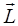, we find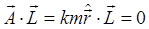, meaning that the constant vectorlies in the plane of the orbit. Next take the dot product ofwith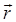, and since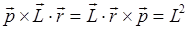, we find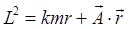, or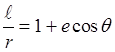where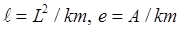and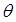is the angle between the planet's orbital position and the Runge Lenz vector. This is the standard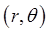equation for an ellipse, withthe semi-latus rectum (the perpendicular distance from a focus to the ellipse), e the eccentricity. Evidentlypoints along the major axis. The point is that the direction of the major axis remains the same: the elliptical orbit repeats indefinitely. If the force law is changed slightly from inverse-square, the orbit precesses: the whole elliptical orbit rotates around the central focus, the Runge Lenz vector is no longer a conserved quantity. Strictly speaking, of course, the orbit isn't quite elliptical even for once around in this case. The most famous example, historically, was an extended analysis of the precession of Mercury's orbit, most of which precession arises from gravitational pulls from other planets, but when all this was taken into account, there was left over precession that led to a lengthy search for a planet closer to the Sun (it didn't exist), but the discrepancy was finally, and precisely, accounted for by Einstein's theory of general relativity. Variation of the Momentum Vector in the Orbit (Hodograph) It's interesting and instructive to track how the momentum vector changes as time progresses, this is easy from the Runge Lenz equation. (Hamilton did this.) From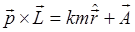, we have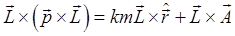That is,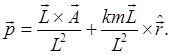Staring at this expression, we see that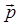goes in a circle of radius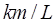about a point distance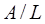from the momentum plane origin. Of course,is not moving in this circle at a uniform rate (except for a planet in a circular orbit), its angular progression around its circle matches the angular progression of the planet in its elliptical orbit (because its location on the circle is always perpendicular to the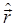direction from the circle center). An orbit plotted in momentum space is called a hodograph. Orbital Energy as a Function of Orbital Parameters Using Runge-Lenz We'll prove that the total energy, and the time for a complete orbit, only depend on the length of the major axis of the ellipse. So a circular orbit and a very thin one going out to twice the circular radius take the same time, and have the same total energy per unit mass. Take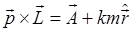and square both sides, giving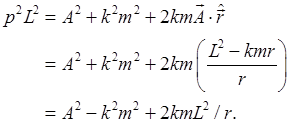Dividing both sides by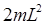,Putting in the values found above,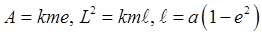we find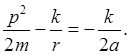So the total energy, kinetic plus potential, depends only on the length of the major axis of the ellipse. Now for the time in orbit: we've shown area is swept out at a rate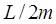, so one orbit takes time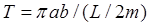, and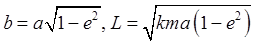, so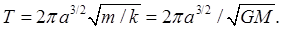This is Kepler's famous Third Law:, easily proved for circular orbits, not so easy for ellipses. Important Hint! Always remember that for Kepler problems with a given massive Sun, both the time in orbit and the total orbital energy/unit mass only depend on the length of the major axis, they are independent of the length of the minor axis. This can be very useful in solving problems. The Runge-Lenz Vector in Quantum Mechanics This is fully discussed in advanced quantum mechanics texts, we just want to mention that, just as spherical symmetry ensures that the total angular momentum and its components commute with the Hamiltonian, and as a consequence there are degenerate energy levels connected by the raising operator, an analogous operator can be constructed for the Runge-Lenz vector, connecting states having the same energy. Furthermore, this raising operator, although it commutes with the Hamiltonian, does not commute with the total angular momentum, meaning that states with different total angular momentum can have the same energy. This is the degeneracy in the hydrogen atom energy levels that led to the simple Bohr atom correctly predicting all the energy levels (apart from fine structure, etc.). It's also worth mentioning that these two vectors, angular momentum and Runge-Lenz, both sets of rotation operators in three dimensional spaces, combine to give a complete set of operators in a four dimensional space, and the inverse-square problem can be formulated as the mechanics of a free particle on the surface of a sphere in four-dimensional space. .u-star-rating-16 { list-style:none; margin:0px; padding:0px; width:80px; height:16px; position:relative; background: url('/.s/t/1211/rating.png') top left repeat-x } .u-star-rating-16 li{ padding:0px; margin:0px; float:left } .u-star-rating-16 li a { display:block;width:16px;height: 16px;line-height:16px;text-decoration:none;text-indent:-9000px;z-index:20;position:absolute;padding: 0px;overflow:hidden } .u-star-rating-16 li a:hover { background: url('/.s/t/1211/rating.png') left center;z-index:2;left:0px;border:none } .u-star-rating-16 a.u-one-star { left:0px } .u-star-rating-16 a.u-one-star:hover { width:16px } .u-star-rating-16 a.u-two-stars { left:16px } .u-star-rating-16 a.u-two-stars:hover { width:32px } .u-star-rating-16 a.u-three-stars { left:32px } .u-star-rating-16 a.u-three-stars:hover { width:48px } .u-star-rating-16 a.u-four-stars { left:48px } .u-star-rating-16 a.u-four-stars:hover { width:64px } .u-star-rating-16 a.u-five-stars { left:64px } .u-star-rating-16 a.u-five-stars:hover { width:80px } .u-star-rating-16 li.u-current-rating { top:0 !important; left:0 !important;margin:0 !important;padding:0 !important;outline:none;background: url('/.s/t/1211/rating.png') left bottom;position: absolute;height:16px !important;line-height:16px !important;display:block;text-indent:-9000px;z-index:1 } Category: Education | Views: 334 | Added by: farrel | | Rating: 0.0/0